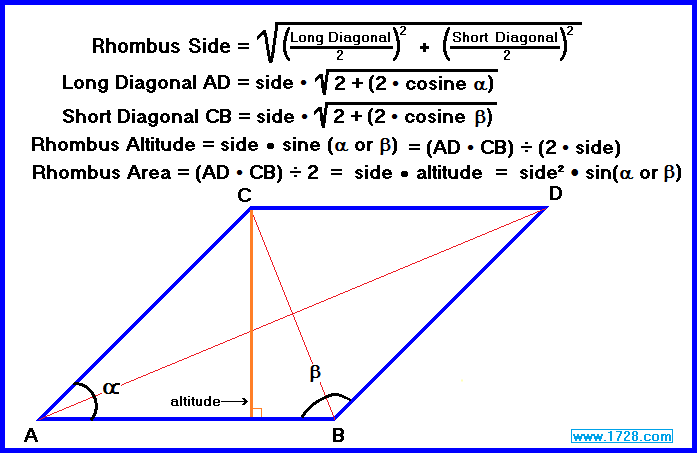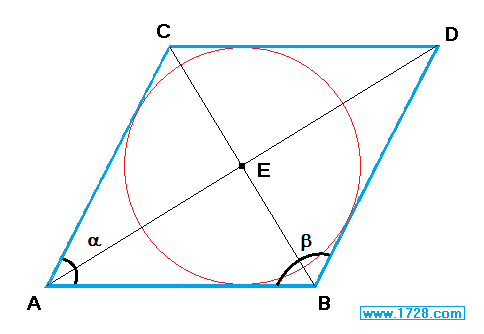Rhombus Calculator
Scroll down for Instructions and Definitions

 Click on the data you know: Side and Angle a Side and Angle b Side and Altitude Long Diagonal AD &       Short Diagonal CBFirst, click on one of the 5 choices, enter the data in the appropriate input boxes, then click "CALCULATE".

Rhombus Facts
All sides are equal.
Lines AD and CB are called diagonals and always meet at right angles.
Line AD is the long diagonal and line CB is the short diagonal.
The diagonals bisect the vertex angles.
Opposite angles are equal.
Every rhombus has 2 acute and 2 obtuse angles.
The altitude (or height) is perpendicular to sides AB & CD.

Area Formulas
Rhombus Area = (AD • CB) ÷ 2

Rhombus Area = side • altitude

Rhombus Area = side² • sin (α or β)

 Inscribing A Circle Within A RhombusAll rhombuses are tangential quadrilaterals, meaning that they are 4 sided figures into which a circle (called an incircle) can be inscribed such that each of the four sides will touch the circle at only one point. (Basically, this means that the circle is tangent to each of the four sides of the rhombus.) To inscribe a circle graphically within a rhombus (using compass and straight edge): • calculate the inradius, which is the altitude ÷ 2 • set the compass precisely to this distance • draw the rhombus diagonals • place the compass point precisely at "E" where the diagonals intersect • Inscribe the circle using point E as its center and the inradius as its radius.

Significant Figures >>>
The default setting is for 5 significant figures but you can change that by inputting another number in the box above.

Answers are displayed in scientific notation and for easier readability, numbers between .001 and 1,000 will be displayed in standard format (with the same number of significant figures.)
The answers should display properly but there are a few browsers that will show no output whatsoever. If so, enter a zero in the box above. This eliminates all formatting but it is better than seeing no output at all.

_____________________